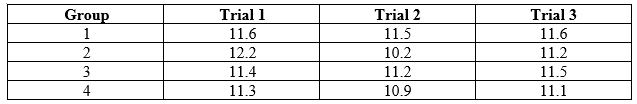# Problem: The density of lead is 11.3 g/cm3. The following table presents lead density data (g/cm3) collected by different groups of students. Determine which of the following groups havea) the most precise data     b) the most accurate data

🤓 Based on our data, we think this question is relevant for Professor Wink's class at UIC.

###### Problem Details

The density of lead is 11.3 g/cm3. The following table presents lead density data (g/cm3) collected by different groups of students. Determine which of the following groups have

a) the most precise data

b) the most accurate dataWhat scientific concept do you need to know in order to solve this problem?

Our tutors have indicated that to solve this problem you will need to apply the Accuracy & Precision concept. You can view video lessons to learn Accuracy & Precision. Or if you need more Accuracy & Precision practice, you can also practice Accuracy & Precision practice problems.

What is the difficulty of this problem?

Our tutors rated the difficulty ofThe density of lead is 11.3 g/cm3. The following table prese...as low difficulty.

How long does this problem take to solve?

Our expert Chemistry tutor, Sabrina took 3 minutes and 21 seconds to solve this problem. You can follow their steps in the video explanation above.

What professor is this problem relevant for?

Based on our data, we think this problem is relevant for Professor Wink's class at UIC.